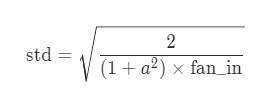# Pytorch Weight Initialisation Layer by layer

#1

I was seeing how the means and standard deviations of the weights change as the model progresses and observed that the standard deviations of the later layers is initilzed much less that the first by default:

Left is the means and right is the stds

This was for a small 4 layer network, even when I made a layer network I observed the same, that the later layers were intilizied to have much lower variance:

As you can see the stds systematically decrease. Can someone explain why this is done? I know we want our ouputs to have mean 0 and std 1 but how is this helping that? I dont think this was covered in the lectures

Code:
Create any CNN in pytorch and then run

``````model=Conv()

def stats(x): return x.mean(),x.std()

for i,e in enumerate(model.children()):
print(i,e)
print('-----Layer Mean and std',stats(e.weight))
``````

# Update

Even with I manually initilise the layers I get the same behavior!

``````model=Conv()

for l in model.children():
try:
init.kaiming_normal_(l.weight)
l.bias.data.zero_()
except:
pass

def stats(x): return x.mean(),x.std()

for i,e in enumerate(model.children()):
print(i,e)
print('-----Layer Mean and std',stats(e.weight))
``````

This is pretty unsettling! pytorch is keeping track of the layer index. Why is this happening?

1 Like

(Yiming Lin) #2

Hi,
Not only “the stds systematically decrease” but also the input channel number of each conv2 layer “systematically increase”.The kaiming_normal_ initialisation method samples the values from a Gaussian distribution whose std is inversely proportional to the input channel number, i.e. the fan_in, by default:Try put conv2d layers with 256 channels at the begining and layers with 32 channels at the end, i.e. decrease the channel number as the network goes deeper. You may observe “the stds systematically increase”2 Likes